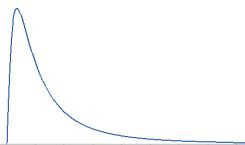# Hyperparameters in Azure Machine Learning

Rating & reviews (0 reviews)
Study Notes

Hyperparameteris a parameter whose value is used to control the learning process. Set HOW the model is trained.
Choosing optimal hyperparameter values for model training is difficult. It must be tuned via Machine Learning mechanisms.
Hyperparameter tuning is accomplished by training the multiple models, using the same algorithm and training data but different hyperparameter values.

Search space= Set of hyperparameter values tried during training experiment.

Hyperparameter types
• Discrete
Hyperparameter values are selected from a particular set of posibilities.
Ex:
Python list
choice([10,20,30])
choice(range(1,100))

Discrete distributions
qnormal
quniform
qlognormal
qloguniform
• Continuus
Can take any value along a scale.
Countinous distributions
nornal
uniform
lognormal
loguniform
Type:
• Discrete hyperparameter (select discrete values from continues distributions)
• qNormal distribution
• qUniformdistribution
• qLognormal distribution
• qLogUniform distribution
• Continuous hyperparameters
• Normal distribution
• Uniform distribution
• Lognormal distribution
• LogUniform distribution
Normal distribution
Normal distribution or Gaussian distribution is a type of continuous probability distribution for a real-valued random variableUniform distribution
Continuous uniform distribution or rectangular distribution is a family of symmetric probability distributions. The distribution describes an experiment where there is an arbitrary outcome that lies between certain bounds.Lognormal distribution
Continuous probability distribution that models right-skewed data.
The lognormal distribution is related to logs and the normal distribution.LogUniform distribution
Continuous probability distribution. It is characterised by its probability density function, within the support of the distribution, being proportional to the reciprocal of the variable.Defining a search space
Create a dictionary with the appropriate parameter expression for each named hyperparameter.
For example, the following search space indicates that the batch_size hyperparameter can have the value 16, 32, or 64, and the learning_rate hyperparameter can have any value from a normal distribution with a mean of 10 and a standard deviation of 3.
from azureml.train.hyperdrive import choice, normal

param_space = {
'--batch_size': choice(16, 32, 64),
'--learning_rate': normal(10, 3)
}

Sampling types
Sampling - how hyperparameters values are selected.
• Grid sampling
Only for discrete hyperparameters
Try every possible combination of parameters in the search space.

from azureml.train.hyperdrive import GridParameterSampling, choice

param_space = {
'--batch_size': choice(16, 32, 64),
'--learning_rate': choice(0.01, 0.1, 1.0)
}

param_sampling = GridParameterSampling(param_space)

• Random sampling
Randomly select a value for each hyperparameter, which can be a mix of discrete and continuous values

from azureml.train.hyperdrive import RandomParameterSampling, choice, normal

param_space = {
'--batch_size': choice(16, 32, 64),
'--learning_rate': normal(10, 3)
}

param_sampling = RandomParameterSampling(param_space)
• Bayesian sampling
Chooses hyperparameter values based on the Bayesian optimization algorithm, which tries to select parameter combinations that will result in improved performance from the previous selection.

from azureml.train.hyperdrive import BayesianParameterSampling, choice, uniform

param_space = {
'--batch_size': choice(16, 32, 64),
'--learning_rate': uniform(0.05, 0.1)
}

param_sampling = BayesianParameterSampling(param_space)

Early termination
Particularly useful for deep learning scenarios where adeep neural network (DNN) is trained iteratively over a number ofepochs
To help prevent wasting time, you can set anearly termination policy that abandons runs that are unlikely to produce a better result than previously completed runs.
The policy is evaluated at an evaluation_interval you specify, based on each time the target performance metric is logged.
You can also set a delay_evaluation parameter to avoid evaluating the policy until a minimum number of iterations have been completed.

Bandit policy
Stop a run if the target performance metric underperforms the best run so far by a specified margin.
from azureml.train.hyperdrive import BanditPolicy

early_termination_policy = BanditPolicy(slack_amount= 0.2,
evaluation_interval=1,
delay_evaluation=5)
This example applies the policy for every iteration after the first five, and abandons runs where the reported target metric is 0.2 or more worse than the best performing run after the same number of intervals.

Median stopping policy
Abandons runs where the target performance metric is worse than the median of the running averages for all runs.
from azureml.train.hyperdrive import MedianStoppingPolicy

early_termination_policy = MedianStoppingPolicy(evaluation_interval=1,
delay_evaluation=5)

Truncation selection policy
Cancels the lowest performing X% of runs at each evaluation interval based on the truncation_percentage value you specify for X.
from azureml.train.hyperdrive import TruncationSelectionPolicy

early_termination_policy = TruncationSelectionPolicy(truncation_percentage=10,
evaluation_interval=1,
delay_evaluation=5)

Running a hyperparameter tuning experiment
Training script must:
• Have an argument for each hyperparameter you want to vary.
• Log the target performance metric
For example, the following example script trains a logistic regression model
• using a --regularization argument to set the regularization rate hyperparameter
• andlogs the accuracymetric with the name Accuracy
import argparse
..
# Get regularization hyperparameter
parser = argparse.ArgumentParser()
args = parser.parse_args()
reg = args.reg_rate
..
..
# calculate and log accuracy
y_hat = model.predict(X_test)
acc = np.average(y_hat == y_test)
run.log('Accuracy', np.float(acc))
...
run.complete()

Configuring and running a hyperdrive experiment
To prepare the hyperdrive experiment, you must use a HyperDriveConfigobject to configure the experiment run
from azureml.core import Experiment
from azureml.train.hyperdrive import HyperDriveConfig, PrimaryMetricGoal

# Assumes ws, script_config and param_sampling are already defined

hyperdrive = HyperDriveConfig(run_config=script_config,
hyperparameter_sampling=param_sampling,
policy=None,
primary_metric_name='Accuracy',
primary_metric_goal=PrimaryMetricGoal.MAXIMIZE,
max_total_runs=6,
max_concurrent_runs=4)

experiment = Experiment(workspace = ws, name = 'hyperdrive_training')
hyperdrive_run = experiment.submit(config=hyperdrive)

Monitoring and reviewing hyperdrive runs
The experiment will initiate a child run for each hyperparameter combination to be tried, and you can retrieve the logged metrics these runs

for child_run in run.get_children():
print(child_run.id, child_run.get_metrics())
# list all runs in descending order of performance
for child_run in hyperdrive_run.get_children_sorted_by_primary_metric():
print(child_run)
# retrieve the best performing run
best_run = hyperdrive_run.get_best_run_by_primary_metric()

References:
Tune hyperparameters with Azure Machine Learning - Training | Microsoft Learn
Lognormal Distribution: Uses, Parameters & Examples - Statistics By Jim
Normal Distribution | Examples, Formulas, & Uses (scribbr.com)